# Rendering a Torus: Geometry, Lighting, and Textures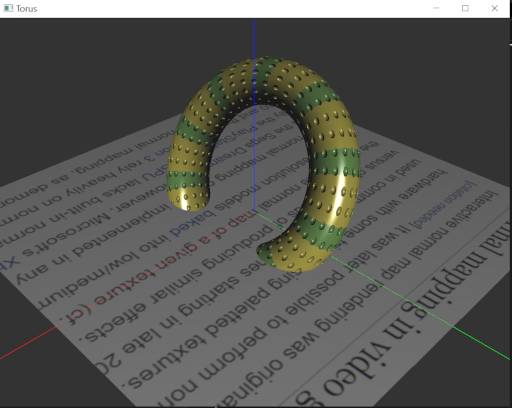# Introduction

“The reports of my death are greatly exaggerated.” -Mark Twain

I’ve been using OpenGL since 1997. In the last two decades, the API has gone through a lot of changes, the most significant being the transition from a fixed function to a programmable pipeline, and the introduction of GLSL (The OpenGL shading Language). Microsoft ditched OpenGL in favour of DirectX many years ago, and Apple followed suit more recently. But despite all this, OpenGL continues to thrive. Game developers at the cutting edge of technology may have moved to other APIs, but OpenGL is still widely used in CAD and 3D Visualization applications, and popular game engines like Unity still support OpenGL. If you are a student learning computer graphics, I’d still recommend that you start with OpenGL.

Vulkan is the new graphics initiative from Khronos, the group behind OpenGL. It’s a high performance, low-level cross-platform graphics API designed to balance CPU and GPU usage. But it is also immensely complex and verbose compared to OpenGL. Even if Vulkan uproots OpenGL completely, I hope for the emergence of some higher level API that doesn’t take all the fun out of graphics programming for beginners.

In this article, I want to go through the process of creating some 3D geometry and using OpenGL and GLSL to render it in various styles.

# Objective

Render a torus using OpenGL, GLSL, and C++, in six different styles:

3. Texture mapping
4. Procedural texture
5. Bump Mapping
6. Rim lighting

I’ll assume that you have some background in C++ and OpenGL. I’ll be mostly focusing on the explanation of the math and graphics techniques. The full code listings can be found in the link in the Downloads section.

Now let’s get started.

# Project Overview

We will use C++ 17 and OpenGL for this project. We will also leverage the following external libraries:

• GLFW - a cross platform OpenGL windowing library.
• glm - an amazing C++ headers-only GLSL compatible library for 3D graphics math.
• stb - a single file image loading library. (Included in repo.)

This project uses CMake for builds. The code is structured as follows:

• src/common contains the following common classes used by the projects:
• Axis3D - draws X/Y/Z axes
• Plane - draws an XY plane of given dimensions
• Render3D - base class for graphics objects
• RenderApp - base class for GLFW based application
• src/torus has the following:
• TorusApp - derives from RenderApp - manages GLFW
• Torus - derives from Render3D - torus rendering code
• main.cpp - creates the TorusApp object

Note that you need to set the following environment variables so your executable can find the shader and texture file directories: NOCG_SHADER_DIR and NOCG_TEXTURE_DIR.

# OpenGL Setup

We will be using OpenGL 4.5 for this project. Here’s the overall rendering strategy, which is typical for modern OpenGL programs.

Setup

2. Create the geometry for the 3D object - vertices, normals, texture coordinates, etc.
4. Create a Vertex Array Object (VAO) for rendering the geometry.
5. Create and configure buffers to hold vertex attribute data.

Rendering

1. Update Projection, View, Model, and Normal matrices as applicable.
2. Enable GLSL program, update uniform data.
3. Render the object using glDrawArrays() or similar calls.

# Torus Geometry

A torus centered at the origin with its axis aligned along +Z can be described by the following set of parametric equations:

\begin{aligned} x &= (R + r \cos{v}) \cos{u} \\ y &= (R + r \cos{v}) \sin{u} \\ z &= r \sin{v} \\ u, v &\in [0, 2\pi] \end{aligned} \tag{1}

In Eq. 1, R is the outer radius of the torus, r is the tube radius. u goes from 0 to , creating the outer ring of the torus, and v goes from 0 to creating the inner tube of the torus.

## Derivation

Since a picture is worth a thousand words, here’s a quick visual proof of the above: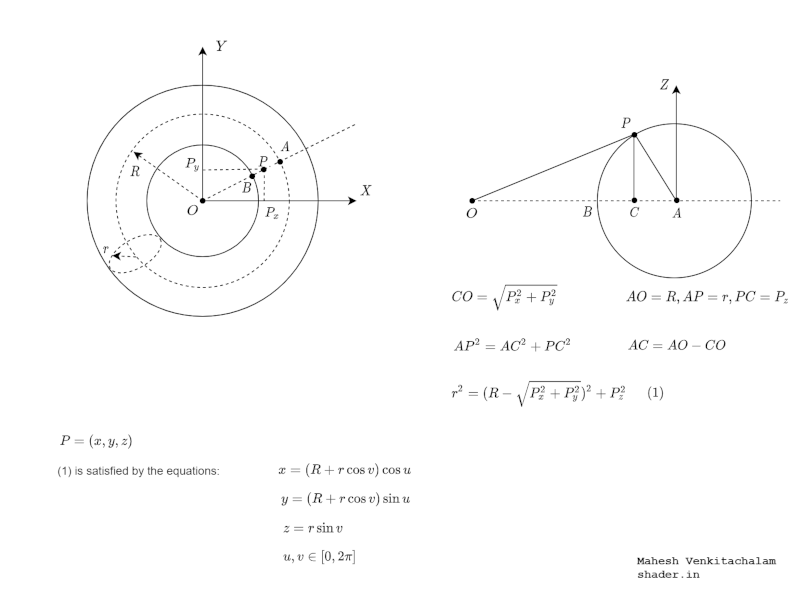The surface normal for a point (x, y, z) on the torus is given by the following set of parametric equations:

\begin{aligned} N_x &= \cos{v} \cos{u} \\ N_y &= \cos{v} \sin{u} \\ N_z &= \sin{v} \end{aligned} \tag{2}

To get some intution on Eq. 2, think about the direction of normals on a circular ring on the torus. They will remain the same even if the ring is translated to the origin. Also, remember that normal at a point P=(x, y) on a unit circle is N=(x, y). So if you set R=0 and r=1 in Eq. 1, you end up with Eq. 2.

The parameters (u, v) form an important coordinate system for rendering our torus. Normalising them gives us texture coordinates - also commonly expressed as (s, t). They are also used in advanced lighting techniues like bump mapping, and that’s where the notion of tangent space comes in.

# Tangent Space

You can think of tangent space as a local coordinate system attached to each point P(x, y, z) on the torus. At this point, there are three vectors that are orthogonal to each other: Tu the tangent along the u direction, Tv the tangent along the v dirtection, and the normal N. This coordinate system is show below.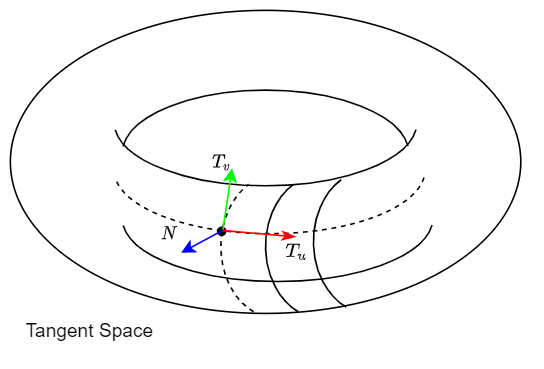Tv is also known as the binormal. Since these vectors are orthogonal to each other, we have:

$T_v = N \times T_u \tag{3}$

It turns out that the tangent space is a convenient coordinate system to store some things - like normals in bump mapping. More on this later.

So we do need the tangent, and it is computed by taking the partial derivative of the surface P as follows:

$T_u = \frac{\partial P(u, v)}{\partial u} \tag{4}$

Computing the above using Eq. 1, we get:

\begin{aligned} T_x &= -(R + r \cos{v}) \sin{u} \\ T_y &= (R + r \cos{v}) \cos{u} \\ T_z &= 0 \\ u, v &\in [0, 2\pi] \end{aligned} \tag{5}

The normalized vectors (N, Tu, Tv) form an orthonormal basis in model space. So if you want convert any point in model coordinates to Tangent space, you would multiply it by the following matrix:

$M = \begin{bmatrix} T_x & T_y & T_z \\ B_x & B_y & B_z \\ N_x & N_y & N_z \end{bmatrix} \tag{6}$

Here, T=Tu, and B=Tv. This matrix is commonly referred to as the TBN matrix.

One thing to be careful about: Be consistent with your coordinate system. If you are operating in world coordinates, you need to convert everything to world coordinates - including M above.

## Rendering the Torus

Now that we have the vertices for the torus, how do we render the surface? Here’s the scheme we’re going to use: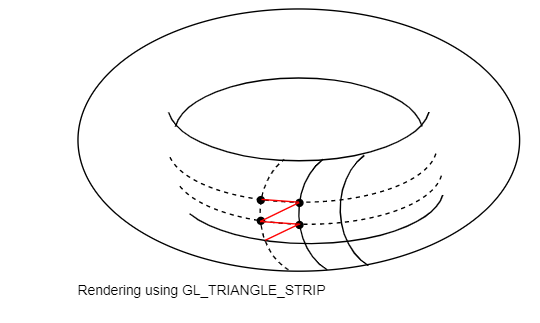We’re going to render the entire torus as a single GL_TRIANGLE_STRIP. The vertex ordering is shown above. Care needs to be taken in closing off the torus in the u and v directions. The last and first set of vertices need to be identical, or you’ll get tears in your geometry due to precision issues. In code, you can do this by using something like i % N to ensure that the vertices roll over at the end.

Here’s the code, from the Torus::_createTorus() method which computes the vertices, normals, and tangents in the order required for rendering. Note that we use std::vector<float> to store the geometry, which is very convenient, and has the same performance as using a C++ array.

# Transforming Normals

Vertices are transformed using the model and view transformations before applying the projection transform. But perhaps surprisingly, Normals do not quite transform the same way. They transform as the transpose of the inverse:

$M_n = (M_v^{-1})^T \tag{7}$

Here, Mn is the normal matrix, and Mv is the modelview matrix. I won’t go into the mathematical dervation of the above, but it’s good to remember that you can use the modelview matrix to transform the normals if the transformations consist of only translations and rotations. (No scaling or shearing.)

Although GLSL has inverse and transpose functions, We will compute Mn in the C++ code and pass it to the shader using a uniform, since we don’t want this (redundant) computation to run on every single vertex in our geometry.

# Lighting Model

Here’s the lighting scheme for our project.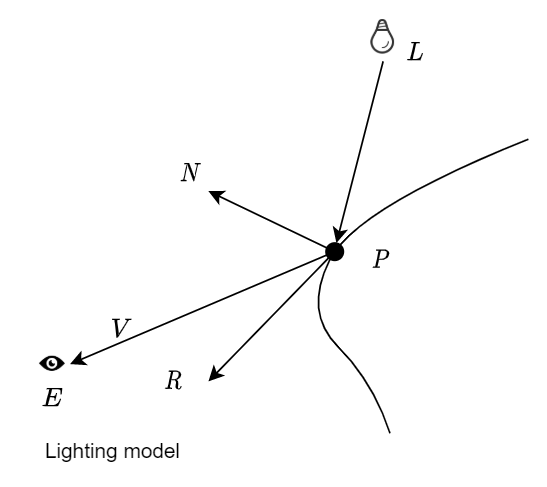In the above figure, L is the light source, P the point on the surface. E the position of the eye, N the normal vector at P, and R the reflection of the light vector about the normal.

We’re going to be computing the final color of a pixel on the surface using the Phong lighting model, given by:

$C = K_a I_a + K_d I_d + K_s I_s \tag{8}$

In the above equation, Ks are a 3-component vectors of the form (r, g, b), and Is are scalar values.

Ka and Ia are the material color and intensity of ambient light. You can think of this as a direction-less contribution of light reflected from surrounding objects.

Ka and Ia are the material color and intensity of diffuse light. This is direction dependent, and Ia can be computed as:

$I_d = L \cdot N \tag{9}$

So the above term is zero when the surface normal is facing away from the light, which makes sense. In the actual code, you would use max(0, L.N) to avoid contributions from negative values of the dot product.

Ks and Is are the material color and intensity of specular lighting. Seen a shiny spot on a ceramic cup that shifts around as you rotate the cup? That’s what we’re trying to simulate.

$I_s = (R \cdot V)^s \tag{10}$

Here, R is the reflection of the light vector about the normal, and V is the eye vector. So the dot product is a measure of how aligned your view is with respect to the light. If you are exactly aligned with the light, the material will appear the most shiny, just as in the real world. The exponent s controls the spread of the specular highlight.

As I mentioned before, it is important in lighting calculations to use a consistent coordinate system. Make sure that you transform all points and vectors to the one you choose - world coordinates, for example.

Now let’s look at specific shading techniques to compute the final color.

In Gouraud shading, we compute Eq.5 in the vertex shader. The color is then passed on to the fragment shader which interpolates it.

Here’s the output: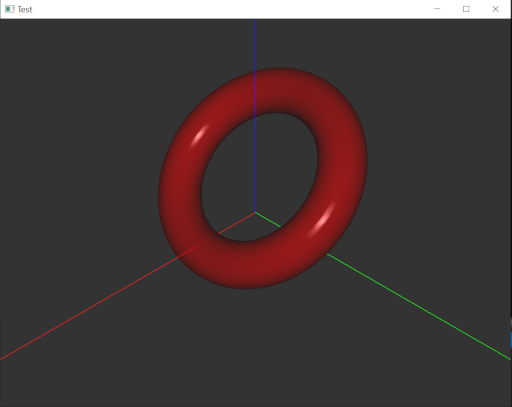Since the color is only computed at the vertices, Gouraud shading misses out on some things. For example, if the lighting causes a shiny spot in the center of a triangle, the interpolation that happens in the fragment shader will miss it. That’s where Phong shading comes in.

In Phong shading, you compute Eg.5 in the fragment shader. N, L and V are computed in the vertex shader and passed on to the fragment shader where they are interpolated.

Here’s the output: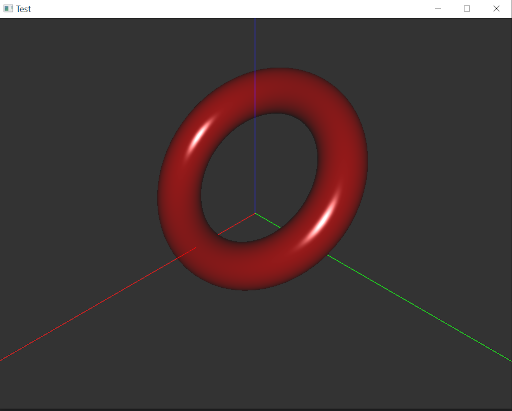The disadvantage of Phong shading is that a lot more computation is done in the fragment shader. So if you are rendering a lot of geometry, you may still prefer Gouraud shading for performance reasons.

# Rim Lighting

Have you noticed that if you take a photo of someone standing in front of a bright window, their outline will show a glow? This effect, also known as rim lighting is used in portrait photography as well. Here’s how we can simulate it using our lighting model.

$I_{rim} = C_r(1.0 - N \cdot V)^p \tag{11}$

Rim lighting happens at the edges of the object. At the edge, normals face away from the eye, and hence the value of the dot product will be small. Subtracting from 1 increases contributions from the edge, which is what we want. The exponent r controls the sharpness, and Cr is the color of the light. Notice that in our model, the position of the light is missing.

You can see above that we’ve used the smoothstep function to avoid sharp transitions in the rim lighting.

The effect of rim lighting is shown below.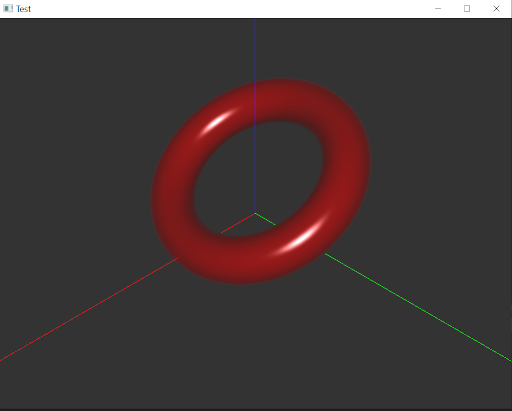# Texture Mapping

For simple texture mapping, we’re just going to load an image and drape it over the torus. Our texture coordinates (s, t) are just the normalized (u, v) coordinates. So (s, t) is in the range [0, 1]. What if you want to tile (repeat) a texture across the torus? Then all you need to do is change the texture coordinates. For example, (4s, 2t) will repeat the texture 4 times in the u direction and 2 times in the v direction. (You also need to ensure that you specify GL_REPEAT when you setup the texture using glTexParameter.)

The vertex shader for the texturing is the same as the one we used for Phong shading. Here’s what the fragment shader for texturing looks like:

The main difference here is how texCol is obtained from the texture sampler, and set as Ka.

Here’s the output: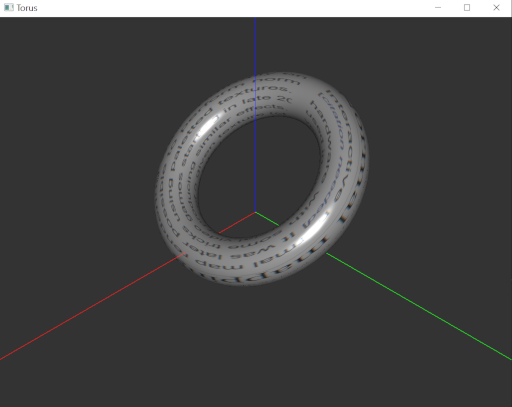# Procedural Textures

Now you know how to texture a torus using an image. But GLSL is very powerful, and gives you the tools to create your own textures in the shader.

Let’s say we want to color our torus with 10 stripes of green along the tube on a background of yellow. How do we go about it?

We need to come up with a periodic function which repeats 10 times along the length. Here’s a possibility:

$f(t) = \sin({20 * 2 \pi t}) \tag{12}$

Here, t is the texture coodinate along the v direction of our torus. Now, we can do a few tweaks like getting rid of negative values, and clamping the values to [0, 1] to give the bands a sharp edge.

Here’s the relevant code from our shader:

By the way, graphtoy is a wonderful tool to experiment with GLSL functions as you prototype ideas for rendering. Here’s a sample output from graphtoy: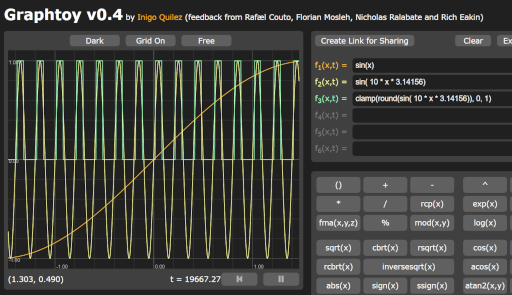And here’s our output: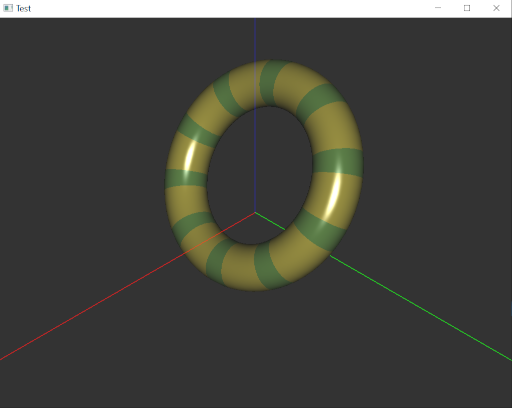# Bump Mapping

Now we come to the most complex style of rendering in this article. Bump mapping, also known as normal mapping, is a trick to give more realism to your graphical objects. It achieves this by tweaking the normals of the object using an image called a bump map.

Remember we talked about tangent space? The normals N in a bump map are in tangent space. We need to convert L, R, and V to tangent space to compute the final color.

But first, we need to create a bump map. Say we want hemispherical bubbles on our torus.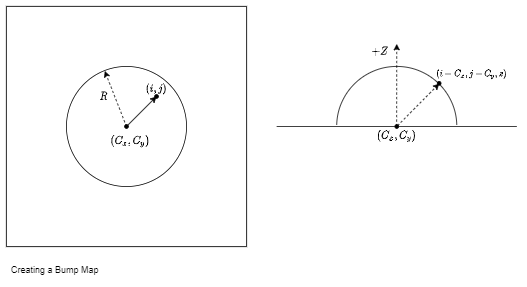The normals on a unit hemisphere centered at origin have are just given by N=(x, y, z). Let C be the center of the image and R be the radius of the bubble in pixels. At any pixel (i, j) on the image you have:

$z = \sqrt{R^2 - ((i-C_x)^2 + (j-C_y)^2)} \tag{13}$

This gives us the normal as:

\begin{aligned} N_x &= (i-C_x)/R \\ N_y &= (j-C_y)/R \\ N_z &= z/R \end{aligned} \tag{14}

One little problem, though. The normal vector components are in the range [-1, 1] where as RGB components in a pixel are in the range [0, 255]. So we encode the normals in the image as follows:

$C_{rgb} = 255(\frac{N_{xyz} + 1.0}{2}) \tag{15}$

This is the reason why normal maps have a bluish color. Most of your normals are in the +Z direction, and so their blue color component will have a higher value compared to the others.

Here’s some Python code which implements the above idea.

And here’s the output from this program: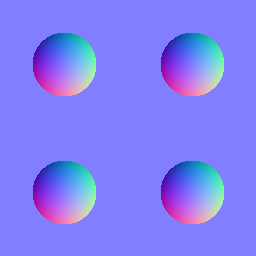Now let’s use this in a shader. Here’s the vertex shader code.

You can see in the above code how the TBN matrix is constructed, and used to transform the light position and the eyepoint. Here’s the fragment shader:

L and V are passed into the fragment shader, and since the normals in the bump map are already in tangent space, all we need to do is look up the values in the texture and use it in the lighting calculations. You can see above that we used vec2(20*fs_in.tc.x, 8*fs_in.tc.y) which repeats the texture 20 times in the u direction and 8 times in the v direction.

And here’s our output: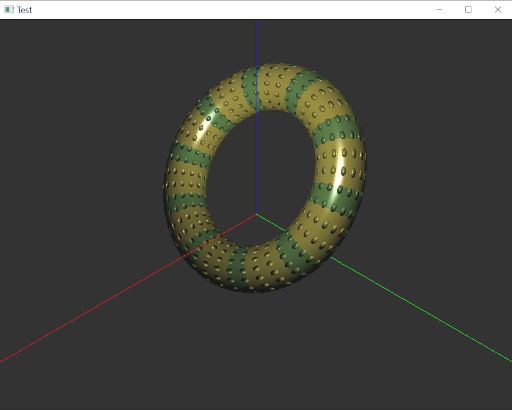# Conclusion

In this article we’ve gone all the way from defining some 3D geometry (a torus), computing the required parameters for lighting it, and rendered it using various styles. Hope you found it interesting!